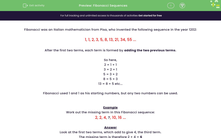# Fibonacci Sequences

In this worksheet, students find the missing term in Fibonacci sequences.Key stage:  KS 3

Curriculum topic:   Algebra

Curriculum subtopic:   Generate Terms of a Sequence

Popular topics:   Algebra worksheets

Difficulty level:#### Worksheet Overview

Fibonacci was an Italian mathematician from Pisa, who invented the following sequence in the year 1202:

1, 1, 2, 3, 5, 8, 13, 21, 34, 55 ...

After the first two terms, each term is formed by adding the two previous terms.

So here,

2 = 1 + 1

3 = 2 + 1

5 = 3 + 2

8 = 5 + 3

13 = 8 + 5 etc...

Fibonacci used 1 and 1 as his starting numbers, but any two numbers can be used.

Example

Work out the missing term in this Fibonacci sequence:

2, 2, 4, ?, 10, 16 ...

Look at the first two terms, which add to give 4, the third term.

The missing term is therefore 2 + 4 = 6

Check that the next terms works too

4 + 6 = 10

6 + 10 = 16

### What is EdPlace?

We're your National Curriculum aligned online education content provider helping each child succeed in English, maths and science from year 1 to GCSE. With an EdPlace account you’ll be able to track and measure progress, helping each child achieve their best. We build confidence and attainment by personalising each child’s learning at a level that suits them.

Get started